Short & Long Answer Questions: Light - Reflection & Refraction

# Short & Long Answer Questions: Light - Reflection & Refraction Notes | Study Science Class 10 - Class 10

## Document Description: Short & Long Answer Questions: Light - Reflection & Refraction for Class 10 2022 is part of Science Class 10 preparation. The notes and questions for Short & Long Answer Questions: Light - Reflection & Refraction have been prepared according to the Class 10 exam syllabus. Information about Short & Long Answer Questions: Light - Reflection & Refraction covers topics like and Short & Long Answer Questions: Light - Reflection & Refraction Example, for Class 10 2022 Exam. Find important definitions, questions, notes, meanings, examples, exercises and tests below for Short & Long Answer Questions: Light - Reflection & Refraction.

Introduction of Short & Long Answer Questions: Light - Reflection & Refraction in English is available as part of our Science Class 10 for Class 10 & Short & Long Answer Questions: Light - Reflection & Refraction in Hindi for Science Class 10 course. Download more important topics related with notes, lectures and mock test series for Class 10 Exam by signing up for free. Class 10: Short & Long Answer Questions: Light - Reflection & Refraction Notes | Study Science Class 10 - Class 10
 1 Crore+ students have signed up on EduRev. Have you?

Ques 1: Which one of the following materials cannot be used to make a lens?
(a) Water

(b) Glass
(c) Plastic
(d) Clay
Ans: (d) Clay

Ques 2: The image formed by a concave mirror is observed to be virtual, erect and larger than the object. Where should be the position of the object?
(a) Between the principal focus and the centre of Curvature
(b) At the centre of curvature
(c) Beyond the centre of curvature
(d) Between the pole of the mirror and Its principal focus.

Ans: (d) Between the pole of the mirror and its principal focus.

Ques 3: Where should an object b. placed In front of a convex lens to get a real
image of the size of the object?
(a) At the principal focus of the lens
(b) At twice the focal length
(c) At infinity
(d) Between the optical centre of the lens and its principal focus

Ans: (b) At twice the focal length.

Ques 4: A spherical mirror and a thin spherical lens have each a focal length of 15 cm. The mirror and the lens are likely to be:
(a) both concave
(b) both convex
(c) the mirror is concave, but the lens is convex
(d) the mirror is convex, but the lens is concave

Ans: (a) Both concave.

Ques 5: No matter how far you stand from a mirror, your image appears erect. The
mirror is likely to be
(a) plane
(b) concave
(c) convex
(d) Either plane or convex

Ans: (d) Either plane or convex.

Ques 6: Which of the following lenses would you prefer to use while reading small letters found in a dictionary?
(a) A convex lens of focal length 50 cm
(b) A concave lens of focal length 50 cm
(c) A convex lens of focal length 5 cm
(d) A concave lens of focal length 5 cm.

Ans: (c) A convex lens of focal length 5 cm.

Ques 7: We wish to obtain an erect image of an object, using a concave mirror of focal length 15 cm. what should be the range of distance of the object from
the mirror? What is the nature of the image? Is the image larger or smaller than the object? Draw a ray diagram to show the image formation in this case.

Ans: We are given the focal length of the concave mirror as f = -15 cm.
For getting an erect image using a concave mirror, the object should be placed at a distance less than the focal length. i.e. 15 cm from the pole. The image formed will be virtual, enlarged and erect.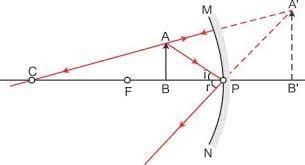Image formation when the object is placed between focus and pole of a concave mirror.

Ques 8: Name the type of mirror used in the following situations.
(a) Headlights of a car
(b) Side/rear-view mirror of a vehicle
(c) Solar furnace

Ans: (a) Concave mirror, to get powerful and parallel beams of light.
(b) Convex mirror because it always gives an erect image and enables the driver to view much larger area.
(c) Concave or parabolic mirror because it can concentrate sunlight at the focus to produce heat in the solar furnace.

Ques 9: One half of a convex lens is covered with a black paper. Will this lens produce a complete image of the object? Verify your answer experimentally. Explain your observations.
Ans: Yes, even when one half of the lens is covered with a black paper, complete image of the object will be formed. Take a convex lens and focus the light from a distant object onto a screen. As expected an image (sharp) is formed at a distance equal to the focal length Cover the lower or the upper half of the lens and focus the light from the same object onto the same screen. You will be able to get a sharp image again; however the brightness of the image will be less in the second case. The same effect w,ll be seen even if the lens is half covered with black strips.

Ques 10: An object 5 cm in length is held 25 cm away from a converging lens of focal length 10 cm. Draw a ray diagram and find the position, size and the nature of the image formed.
Ans: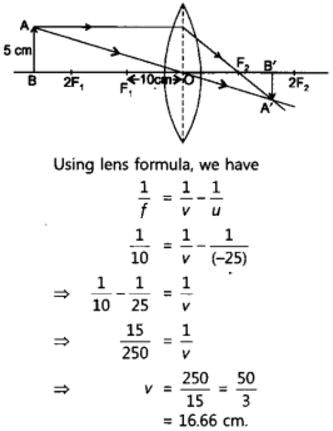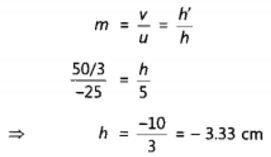Therefore, the image is formed between F2 and 2F2 on the other side of the lens. It is real and inverted, and smaller in size than the object.

Ques 11: A concave lens of focal length 15 cm forms an image 10 cm from the lens. How far is the object placed from the Pens? Draw the ray diagram.
Ans: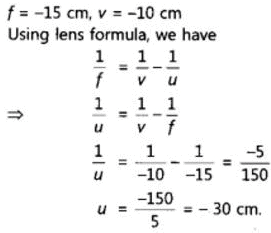Therefore the object  is placed at 30 cm from the lens. The ray diagram is given as follows: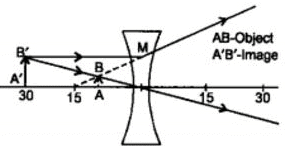Ques 12: An object is placed at a distance of 10 cm from a convex mirror of focal length 15 cm. Find the position and nature of the image.
Ans: f = +15 cm. u = -1o cm
For mirror, we have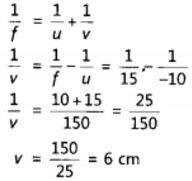The image must be virtual and erect.

Ques 13: The magnification produced by a plane mirror is +1. What does this mean?
Answer: This means that size of the image is equal to the size of the object.

Ques 14: An object 5.0 cm in length is placed at a distance of 20 cm in front of a convex mirror of radius of curvature 30 cm. Find the position of the image nature and size.
Ans: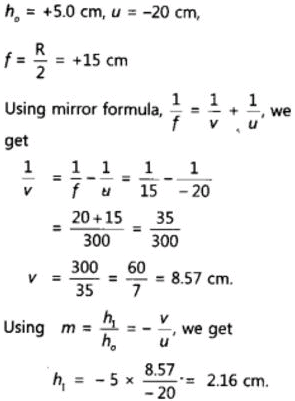Since v is +ve the image is virtual. Since h1 = 2.16cm < 5.0 cm, the image is diminished.

Ques 15: An object of size 7.0 cm is placed at 27 cm in front of a concave mirror of focal length 18 cm. At what distance from the mirror should a screen be placed, so that a sharp focused image can be obtained? Find the size and the nature of the image.
Ans: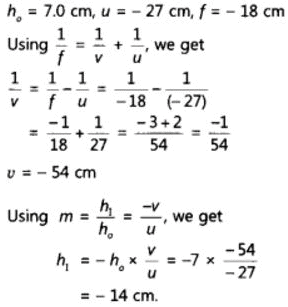Since h1 > h0 the image is enlarged. As the value of h1 is -ve, the image is inverted. Since v is -ve, the image is real.

Ques 16: Find the focal length of a lens of power -2.0 D. What type of lens is this?
Ans: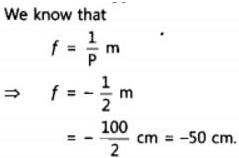As the local length of lens is -ve, it will be a concave lens.

Ques 17: A doctor has prescribed a corrective lens of power +1.5 D. find the focal length of the lens. Is the prescribed lens diverging or converging?
Ans: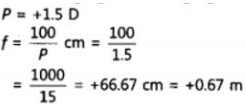As the focal length is +ve it is convex lens. Hence, it is a converging lens.

The document Short & Long Answer Questions: Light - Reflection & Refraction Notes | Study Science Class 10 - Class 10 is a part of the Class 10 Course Science Class 10.
All you need of Class 10 at this link: Class 10

## Science Class 10

83 videos|341 docs|162 tests
 Use Code STAYHOME200 and get INR 200 additional OFF

## Science Class 10

83 videos|341 docs|162 tests

Track your progress, build streaks, highlight & save important lessons and more!

,

,

,

,

,

,

,

,

,

,

,

,

,

,

,

,

,

,

,

,

,

;